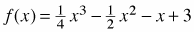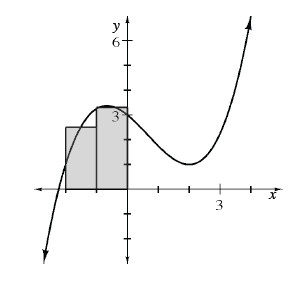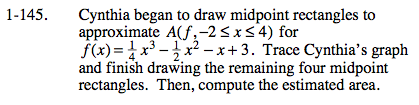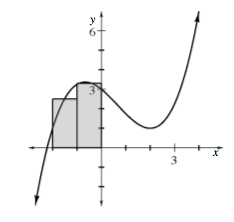### Home > CALC > Chapter 1 > Lesson 1.4.1 > Problem1-145

1-145.
1. Cynthia began to draw midpoint rectangles to approximate A(f, −2 ≤ x ≤ 4) for. Trace Cynthia's graph and finish drawing the remaining four midpoint rectangles. Then, compute the estimated area. Homework Help ✎Make the remaining rectangles. Then calculate the areas of each rectangle and add them together to find A(f, −2 ≤ x ≤ 4).

The width of all of the rectangles is 1.